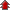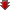# Differential Equations

• NCERT Chapter### Exercise 1

•  Q1 Determine order and degree(if defined) of differential equation \begin{align} \frac{d^4y}{dx^4}\;+\;\sin(y^m)\;=0\end{align} Q2 Determine order and degree(if defined) of differential equation y' + 5y = 0 Q3 Determine order and degree(if defined) of differential equation \begin{align}\left(\frac{ds}{dt}\right)^4\;+\;3s\frac{d^2s}{dt^2}\;=\;0\end{align} Q4 Determine order and degree(if defined) of differential equation \begin{align}\left(\frac{d^2y}{dx^2}\right)^2\;+\;cos\left(\frac{dy}{dx}\right)\;=\;0\end{align} Q5 Determine order and degree(if defined) of differential equation \begin{align}\frac{d^2y}{dx^2}=\cos3x  + sin3x\end{align} Q6 Determine order and degree(if defined) of differential equation (ym)2 + (yn)3 + (y')4 + y5 =0 Q7 Determine order and degree(if defined) of differential equation ym + 2yn + y' =0 Q8 ' + y =ex">Determine order and degree(if defined) of differential y' + y =ex Q9 Determine order and degree(if defined) of differential equation yn + (y')2 + 2y =0 Q10 Determine order and degree(if defined) of differential equation yn + 2y' + siny = 0 Q11 The degree of the differential equation \begin{align}\left(\frac{d^2y}{dx^2}\right)^3\;+ \left(\frac{dy}{dx}\right)^2+\;sin\left(\frac{dy}{dx}\right)\;+ 1=\;0\end{align} is (A) 3 (B) 2 (C) 1 (D) not defined Q12 The order of the differential equation \begin{align}2x^2\frac{d^2y}{dx^2}\;- \;3\frac{dy}{dx}\;+ y=\;0\end{align} is (A) 2 (B) 1 (C) 0 (D) not defined### Exercise 2

•  Q1 y = ex +1 : yn -y' = 0 Q2 y = x2 + 2x + C : y' - 2x - 2 = 0 Q3 y = cosx + C : y' + sinx = 0 Q4 \begin{align} y= \sqrt{1+x^2} : y^{'}=\frac{xy}{1+x^2}\end{align} Q5 ≠ 0)">y = Ax : xy' = y (x ≠ 0) Q6 \begin{align} y = xsinx:xy{'}=y +x\sqrt{x^2 -y^2}(x\neq0\; and\; x>y\; or\; x<-y)\end{align}